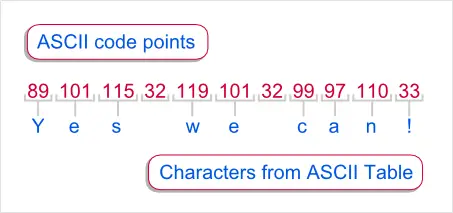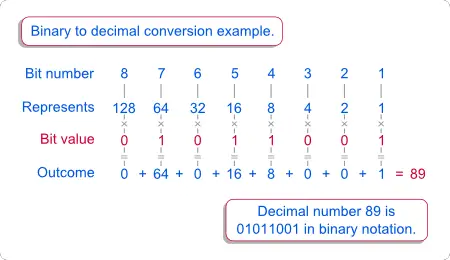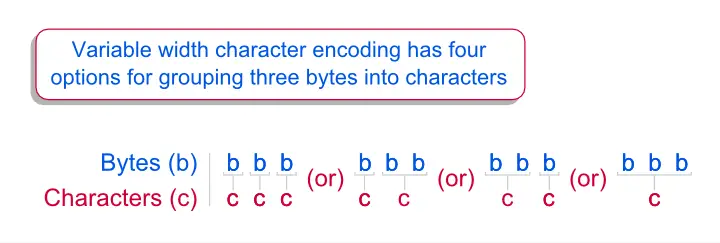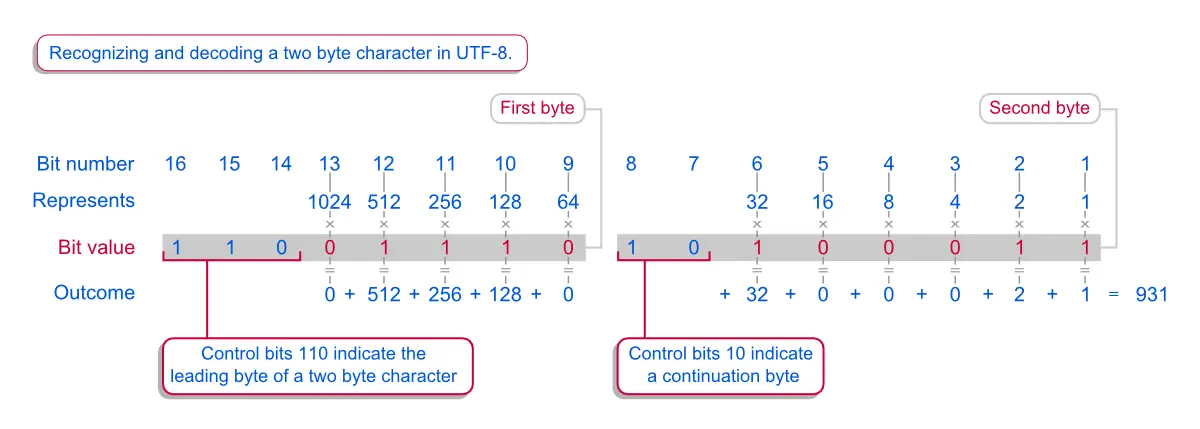# SPSS tutorials

BASICS DATA ANALYSIS T-TEST ANOVA CHI-SQUARE TEST

# Unicode

## Introduction

• You may have heard the phrase that "computers only process ones and zeroes". These are formally referred to as bits.
• This raises the question how computers represent letters (like Y, e and s).
• Representing characters using only bits basically involves two steps. First, each letter is represented by a number. Second, each number is represented by bits using binary notation.
• We'll first discuss how letters are converted to numbers. Next, we'll introduce binary notation.

## ASCIILetters Represented by ASCII Numbers
• For representing letters by numbers, early computer scientists started by writing down a list of all the letters and special characters they wanted to use.
• Next, they simply numbered this list (starting from zero). Like so, each letter was assigned its own (unique) number. (Such numbers are formally referred to as code points.) The outcome of this exercise is known as the ASCII table.
• ASCII consists of 128 code points, each representing some character. These include all lower and upper case letters, the digits 0 through 9 and some special characters such as : and -. Note that English text is usually restricted to these 128 characters. An exception are words with diacritical marks such as résumé or façade.
• From the ASCII table it can be seen that - for example - "Y" is written as 89 and "e" as 101.
• The ASCII table also holds some non printing characters such as space (code point 32), tab (9) and carriage return (13). Most other non printing characters may look less familiar. Many of these have been long since out of use.
• The illustration above shows how the phrase "Yes we can!" is written in ASCII code points.

## Binary NotationBinary Notation Example
• This tutorial began with the question how letters can be represented by bits ("ones and zeroes"). The first step is to represent each character by a code point (remember this is just a number), possibly by using the ASCII table.
• The second step is to represent code points by bits. This is referred to as binary notation.
• The illustration shows how the letter "Y" (ASCII code point 89) is written as `0101 1001` in binary notation.
• As illustrated, every bit represents some number. The first bit (counting from right to left) represents the number 1. The second bit represents 2. The third and fourth bits represent 4 and 8.
• Each of these numbers (1, 2, 4, 8, 16 and so on) may or may not be switched on by its corresponding bit. It's on if its bit is 1 and off if its bit is 0.
• The sum of all numbers that are switched on returns the code point that is represented by the bits. Lastly, the character for this code point is fetched from the ASCII table.

## Code Pages

• The ASCII table may seem to make perfect sense but there's something awkward about it. Remember that bits are usually organized in groups of 8. Such groups of 8 bits are commonly referred to as bytes.
• One byte can represent (28=) 256 different values (0 through 255). One peculiarity of ASCII is that it uses only 128 different characters (0 through 127).
• Technically, this means that ASCII only uses the last 7 bits of each byte. The first bit (representing 128) is always zero. This may seem trivial but we'll explain the importance of this a bit later on.
• Now what about the remaining 128 code points (128 through 255) that a single byte can represent?
• Basically, different languages use these extra characters in different ways. For example, the Spanish use the ¿ character. Since it is not included in the ASCII table, it was assigned to one of the code points that ASCII doesn't use.
• However, other languages needed other characters (such as ô in French or ë in Dutch). Like so, different languages extended the ASCII table in different ways.
• Such extended character tables are formally known as code pages, the best known of which is Windows-1252, somewhat incorrectly referred to as ANSI.

## Problems with Code Pages

• In short, a single byte code page is simply a list of code points (0 through 255) with a normal language character assigned to each of them.
• Under the hood, each character you type is replaced by one of the code points from the code page you're using. So the actual contents of a text file is just a huge sequence of such numbers. And when you open one, the process is reversed. Each number is replaced by a character from the code page you're using.
• Now say my Spanish friend writes "¿Que tal?". His computer uses a Spanish code page in which the ¿ is represented by 128.
• He sends this as a text file to me but my computer uses a Dutch code page in which 128 represents "ë". So on opening the text file, I'll see "ëQue tal?"
• Since the Dutch and Spanish code pages are reasonably similar, this problem is limited. But perhaps I also receive some text encoded using a Russian code page. Now if my computer decodes this using a Dutch code page, the text will show up as an incomprehensible mix of random characters.
• These examples demonstrate that different computers using different code pages may complicate the exchange of text. Ideally, all computers would use a single, universal code page. Such a code page has been built over the past years and is referred to as Unicode.

## Unicode

• Unicode is basically a huge list consisting of all characters used in all languages. All characters have a code point (starting from zero) by which they can referenced.
• In contrast to code pages, not all characters in Unicode are represented by a single byte. Remember that one byte can represent 256 different characters. This is not nearly sufficient for the 110,000+ characters making up Unicode.
• An easy solution for this seems using three bytes for each character. (Note that two bytes can represent 65,536 characters which is still not sufficient for all characters in Unicode.)
• However, this has two disadvantages. The first is that a lot of file sizes will triple if every character needs 3 bytes instead of 1. Note that web pages also consist mostly of text so this would substantially increase the pressure on the network capacity of the internet.
• Second, all existing text that was once encoded using a single byte code page can't be represented at all by a three byte code page. This incompatibility arises because three letters in the original document (three bytes) would now show up as a single letter (three bytes).
• However, a brilliant solution was invented that solves both problems. It is called Universal Transformation Format - 8 bit, commonly abbreviated to UTF-8.

## UTF-8

• The essence of UTF-8 is that each character may be represented by either one, two or more bytes. This is called variable width character encoding: the number of bytes representing each character differs between characters.
• But consider the following. A text file containing three bytes is opened. Do these three bytes represent three separate characters? Possibly. But they could also represent a single three byte character. Or one two byte character followed by a single byte character (or reversely). The figure below illustrates the problem.Three Bytes can Represent Characters in Four Ways
• So how can a text editor know how to interpret three bytes? The trick here is that some of the bits in each byte are reserved to indicate how bytes are grouped into characters. We'll call these control bits. Only the remaining bits are converted into a decimal number - for which the corresponding character is then fetched from Unicode table.

## UTF-8 - How Does it Recognize Characters?UTF-8 Character Encoding Illustration
• The figure above illustrates how a two byte character is recognized as such and decoded. (It returns 931 which is the Unicode number corresponding to Σ.) This uses three patterns which we'll explain below.
• Any byte starting with a zero indicates a single byte character. The first bit is a control bit and merely indicates that this byte represents a character by itself. We'll describe this pattern as `0??? ????`.
• Now remember that the 128 ASCII characters follow this exact same pattern. Therefore, these 128 characters could be used as the first 128 characters in Unicode using the exact same (single) bytes. The result is that any text ever written in ASCII will show up just fine when it's decoded according to Unicode. That is, UTF-8 is compatible with ASCII.
• A second consequence is that most text using the Latin alphabet - especially English - needs only a single byte per character. This keeps file sizes small and thus reduces network traffic.
• Another pattern is `11?? ????`. This indicates the leading byte of a multibyte character. The number of ones at the start indicates how many bytes make up this character.
• The third pattern is `10?? ????`. This indicates a continuation byte in a multibyte character.
• Note that the three byte patterns used in UTF-8 automatically imply some extra validity checks. For example, `0??? ????` can never be followed by `10?? ????`. The first byte is a single byte character so the second byte can't be a continuation byte. Likewise, `111? ????` must always be followed by exactly two continuation bytes.

# Let me know what you think!

*Required field. Your comment will show up after approval from a moderator.

# This tutorial has 2 comments

•### By K Prem Kiran on July 12th, 2018

Amazing. Wonderfully written

•### By Hezy on April 20th, 2015

You have done good jobs!!!!!!!!!!!!! bravo